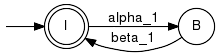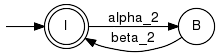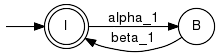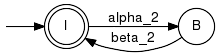# Synchronous Composition

Given two discrete event systems, the synchronous composition is a discrete event system that models the respective component behaviour under the constraint that shared events must be executed synchronously. Thus, each component restricts the behaviour of the other component.

In terms of languages L1 and L2 over alphabets Sigma1 and Sigma2, respectively, the resulting behaviour is defined

L_res = L1 || L2 := Pinv1(L1)  Pinv2(L2) = {s  Sigma*| P1(s)  L1 and P2(s)  L2},

where Pinv1 and Pinv2 denote the set-valued inverse of the natural Projections P1:Sigma*Sigma1*, P2:Sigma*Sigma2*, Sigma = Sigma1  Sigma2, respectively.

Note. If the alphabet Sigma1 is empty, we have either L1 = 0 or L1 = {epsilon}. In this case, the synchronous composition technically evaluates to L_res = 0 or L_res = L2, respectively. A similar line of thought applies to the situation where Sigma2 is empty.

Note. Functions in this section maintain event attributes, provided that they match for all arguments.

## Parallel

Computes the parallel composition of two or more generators.

##### Signature:

Parallel(+In+ Generator G1, +In+ Generator G2, +Out+ Generator GRes)

Parallel(+In+ GeneratorVector GVec, +Out+ Generator GRes)

Parallel(+In+ Generator G1, +In+ Generator G2, +Out+ ProductCompositionMap CompMap, +Out+ Generator GRes)

##### Detailed description:

Given two generators G1 and G2, this function computes the parallel composition GRes = G1 || G2, with

L(GRes) = L(G1) || L(G2)
Lm(GRes) = Lm(G1) || Lm(G2)

The resulting generators alphabet is the union of the argument alphabets.

Technically, Parallel constructs its result on the product of the state spaces of G1 and G2, i.e. Q_res = Q1 x Q2. Thus, to a resulting state q = (q1,q2) correspond the component states q1 and q2. Transitions are enabled in GRes whenever they are enabled in each component that include the event in their respective alphabet:

((q1,q2), o, (q1',q2'))  delta_res
o  Sigma1  or  (q1, o, q1')  delta1 and
o  Sigma2  or  (q2, o, q2')  delta2

There are two more variants of Parallel:

• when called with an vector valued input argument, the overall synchronous composition is computed by repetetive invocation of the standard routine;

• an optional output argument can be supplied to record a so called composition map, which relates the states of the result and the states of the arguments.

##### Example:

Our first example composes two independent "very simple machines". Since the components do not share any events, the parallel compositions turns out as the so called shuffle product.

 Component G1 Component G2Composition G=G1 || G2##### Example:

In this example, the shared events are {a, b}. Component G2 does not impose any restrictions on the generated language, i.e. Pinv2(L(G2)) = Sigma*. However, G2 requires the marked language not to end with a b-event (when projected to Sigma2).

 Component G1 Component G2Composition G=G1 || G2##### Parameter Conditions:

In this implementation, only accessible states are generated. On deterministic input this functions constructs a deterministic output. The resulting generators controllability attributes are copied from the arguments. It is considered an error if the controllability flags in the arguments don't match.

## IsNonblocking

Test a generator/ two languages for being nonblocking.

##### Signature:

IsNonblocking(+In+ Generator G, +Out+ Boolean BRes)

IsNonblocking(+In+ Generator G1, +In+ Generator G2, +Out+ Boolean BRes)

IsNonblocking(+In+ GeneratorVector G, +Out+ Boolean BRes)

##### Detailed description:

An individual discrete event system modelled by a generator G may block in the sense that it attains a state from which no marking can be reached. Technically, G is said to be non-blocking, if

L(G) = Closure(Lm(G)) .

The above equality requires that any string generated by G can be extended to a marked string. For deterministic generators, an equivalent condition is that any state that is accesible must also be co-accessible. This condition is tested by the function IsNonblocking.

The synchronous composition of two discrete event systems modelled by the generators G1 and G2 may block in the sense that the one system prevents the other satisfying its acceptance condition. Formally, two languages are non-conflicting, if

Closure( L1||L2 )  =  Closure(L1) || Closure(L2) .

Here, the righthand side is interpreted as a composition where the synchronisation is performed on a per event basis, without considering markings. The equality then guarantees that a marking can allways be reached.

When applied to two generators, the function IsNonblocking first performs the Parallelcomposition and then tests, whether the resulting generator is non-blocking. Provided that the two individual generators are non-blocking, i.e.

L(G1) = Closure(Lm(G1)) and
L(G2) = Closure(Lm(G2)) ,

the test returns true iff

Closure( Lm(G1)||Lm(G2) )  =  Closure(Lm(G1)) || Closure(Lm(G2)) .

When a large number of generators is to be tested for conflicts, performing the overall parallel composition may not be practical. The literature [C4] addresses such situations and proposes various conflict-equivalent abstraction methods to be applied to the individual systems before composition. These methods are used when invoking IsNonblocking with the GeneratorVector argument. However, our implementation is still experimental and does not allways achieve the expected performance.

##### Parameter Conditions:

For blocking in the sense of accessible but not co-accessible states, no restrictions apply. For the language based notion of conflicts, the individual generators are must be deterministic and non-blocking for a sufficient and necessary test.

## OmegaParallel

Parallel composition with relaxed acceptance condition.

##### Signature:

OmegaParallel(+In+ Generator G1, +In+ Generator G2, +Out+ Generator GRes)

##### Detailed description:

The marking introduced by the Parallel composition requires that each component accepts a string simultaneously. This condition may turn out too restrictive, in particular when focus is on the infinite time behaviour. The function OmegaParallel relaxes the acceptance condition, in that it requires each component always to eventually accept the generated prefix, but not necessarily simultaneously with the other component:

L(GRes) = L(G1) || L(G2)
Bm(GRes) = Bm(G1) || Bm(G2)
:= {w  Sigma^w| P1(w)  Bm(G1) and P2(w)  Bm(G2) }

where Bm(G) denotes the omega language realised by G with Buechi acceptance condition, see Generator.

Technically, OmegaParallel constructs its result on the product of the state spaces of G1 and G2 with an additional boolean flag. The flag is used to mark those states in GRes that correspond to a G2-marking with most recent marking in G1. Thus, a trajectory that passes a GRes-marking infinitely often, also passes infinitely many marked states in G1 and G2.

##### Example:
 Component G1 Component G2Composition G=G1 ||| G2##### Parameter Conditions:

In this implementation, only accessible states are generated. Arguments are required to be deterministic, and so will be the result. It is considered an error if the controllability flags in the arguments don't match.

## Product

Computes the product composition of two genertors.

##### Signature:

Product(+In+ Generator G1, +In+ Generator G2, +Out+ Generator GRes)

Product(+In+ Generator G1, +In+ Generator G2, +Out+ ProductCompositionMap CompMap, +Out+ Generator GRes)

##### Detailed description:

Given two generators G1 and G2, this function computes the product composition GRes = G1 x G2, with

L(GRes) = L(G1)  (G2)
Lm(GRes) = Lm(G1)  Lm(G2)

The resulting generators alphabet is the intersection of the argument alphabets.

Technically, Product constructs its result on the product of the state spaces of G1 and G2, i.e. Q_res = Q1 x Q2. Thus, to a resulting state q = (q1,q2) correspond the component states q1 and q2. Transitions are enabled in GRes whenever they are enabled in both components.

((q1,q2), o, (q1',q2'))  delta_res
(q1, o, q1')  delta1 and
(q2, o, q2')  delta2

An optional output argument can be supplied to record a so called composition map, which relates the states of the result and the states of the arguments.

##### Parameter Conditions:

In this implementation, only accessible states are generated. On deterministic input this functions constructs a deterministic output. The resulting generators controllability attributes are copied from the arguments. It is considered an error if the controllability flags in the arguments don't match.

libFAUDES 2.28b --- 2019.12.01 --- with "synthesis-observer-diagnosis-iosystem-hiosys-multitasking-coordinationcontrol-timed-iodevice-simulator-luabindings"